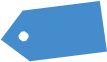# Dow Trendline Termination

This is my first experimental video. I have been a member of Slope family since 2009, and finally got some guts to do a rough video. Criticisms are welcome. In the video the point calculations are not accurate, as it was just for the concept.

GREEN LINE:

June 24-July 25= 31 days

14548-15617=1069 points
(1069/31=35.633. avg. points move per day)

BLACK LINE:
July 10-July 24=14 days

15255-15617=362 points
(362/14= 25.8571 avg. points move per day)

ORANGE DOTED LINE:
July 26-Aug 05= 11 days

15400-15651=251 points
(251/11= 22.8181 avg. points move per day)

RED LINE: (steeper angle than orange line)

Jul 31-Aug 02 = 2/3 days (Aug 2nd was the new high)

15485-15650= 165 points
(July 31 high was 15635), so 15650-15635=15

points extra from previous high

(165/2=82.5 points avg. move per day.)
(165/3= 55 points avg. move per day.)

THIN BLUE LINE:(steeper angle then red line)

Aug 02-Aug 04= 2/3 day

15550-15654= 104 points
(Aug 1st high was 15650, so 15654-15650=4 extra

points from previous high)

(104/2=52 points avg. move per day.)
(104/3=34.66 points avg. move per day.)

Larger trend total avg. move:
june 24-Aug 2=39 days

15650-14548= 1102 points

(1102/39=28.2564 avg points move per day)